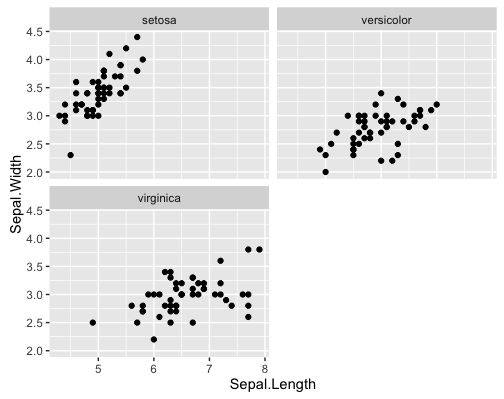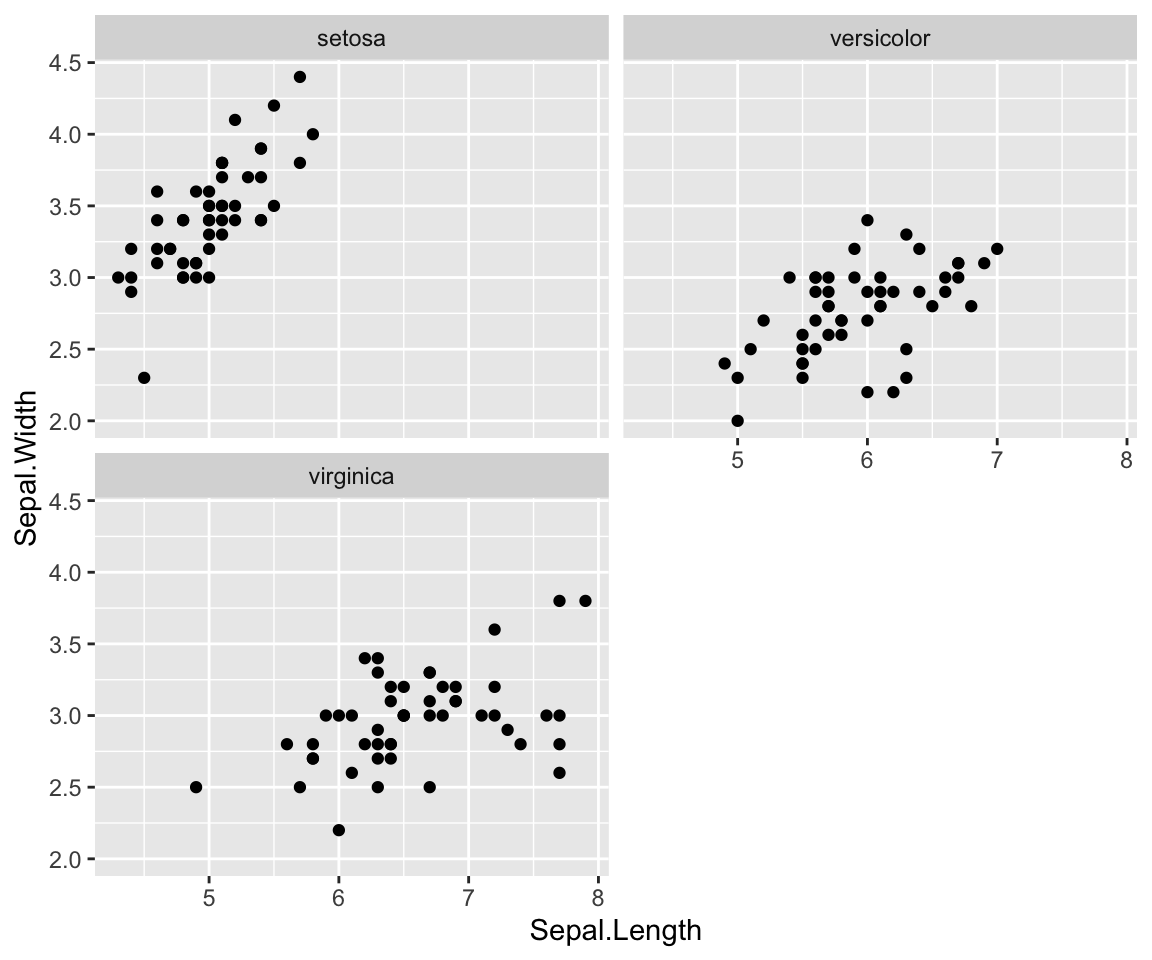One of the most annoying features of ggplot2 was the missing x-axis scale on facet-plots with some shorter columns.

To illustrate the problem see the following (pre-2.2-version) example:

 ``````1 2 3 4 5 `````` ``````library(ggplot2) ggplot(data=iris, aes(x=Sepal.Length, y=Sepal.Width)) + geom_point() + facet_wrap(~Species, nrow=2) ``````As you can see the scale of the x-axis under the second, shorte column is missing.

But with version 2.2.0 of ggplot2 this problem is solved:If you ask how to install an older version of a package:

 ``````1 2 `````` ``````library(devtools) install_version("ggplot2", version = "2.1.0", repos = "http://cran.r-project.org") ``````